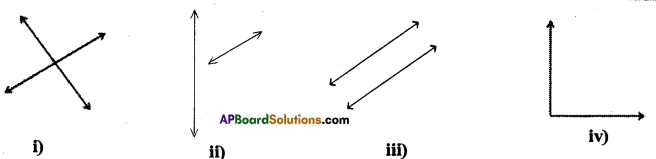# AP Board 7th Class Maths Solutions Chapter 4 Lines and Angles Ex 1

AP State Syllabus AP Board 7th Class Maths Solutions Chapter 4 Lines and Angles Ex 1 Textbook Questions and Answers.

## AP State Syllabus 7th Class Maths Solutions 4th Lesson Lines and Angles Exercise 1Question 1.
Name the figure drawn below.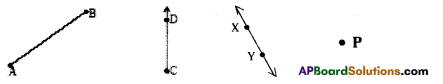Solution:
Line segment $$\overline{\mathrm{AB}}$$
Ray $$\overline{\mathrm{DC}}$$
Line $$\overline{\mathrm{SY}}$$
Point P

Question 2.
Draw the figures for the following.
(i) $$\overline{\mathrm{OP}}$$
(ii) Point X
(iii) $$\overline{\mathrm{RS}}$$
(iv) $$\overline{\mathrm{CD}}$$
Solution: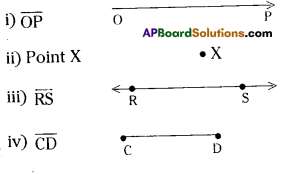Question 3.
Name all the possible line segments in the figure.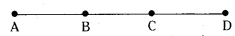Solution:
$$\overrightarrow{\mathrm{AB}}, \widehat{\mathrm{BC}}, \overline{\mathrm{CD}}, \overline{\mathrm{AC}}, \overline{\mathrm{AD}}, \overline{\mathrm{BD}}$$

Question 4.
Write any five examples of angles that you have observed arround.
Example: The angle formed when a scissor is opened.
Solution:
i) Angle formed when a door is opened.
ii Angle between two adjacent edges of a blackboard.
iii) Angle between two adjacent edges of a ruler.
iv) Angle between two adjacent edges of a set square.
v) Angle formed at elbow.Question 5.
Identify the following given angles as acute, right or obtuse.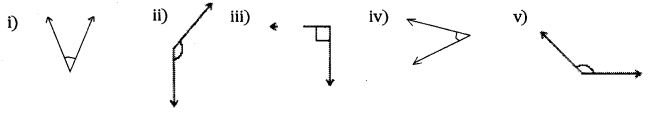Solution:
i) acute
ii) obtuse.
iii) right
iv) acute
v) obtuse.Question 6.
Name all the possible angles you can find in the following figure. Which are acute, right, obtuse and straight angles?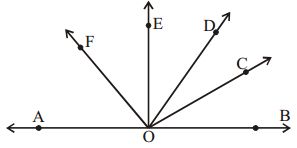Solution:
Acute angles = ∠AOF,∠FOE, ∠EOD, ∠DOC, ∠COB, ∠EOC, ∠DOB, ∠DOF
Obtuse angles = ∠AOD, ∠AOC, ∠BOF
Right angle = ∠AOE,∠BOE

Question 7.
Which of the following pairs of lines are parallel? Why?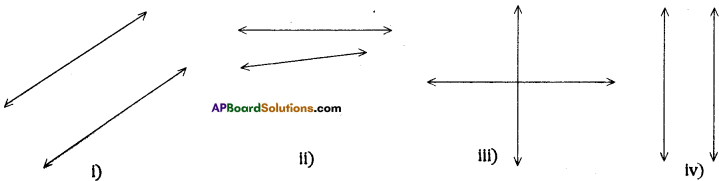Solution:
Figure (i) and (iv) are pairs of parallel lines because however long they are produced, they
never intersect each other.Question 8.
Which of the following lines are intersecting?